# How to Transpose Rows and Columns in Excel

This post will guide you how to convert row data to columns in Excel. How do I convert columns to rows with Paste feature in Excel 2013/2016.

## Convert Rows to Columns Using Paste Special

Assuming that you have a list of data in range A1:D5, and you want to change columns to rows for your data in the current worksheet in Excel. You can use the Paste Special feature to achieve the result. Just do the following steps:

#1 select the range of cells that you want to transpose.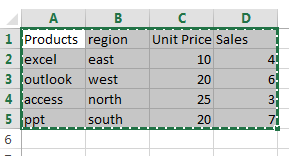#2 press Ctrl + C keys on your keyboard to copy the selected cells.

#3 select one black cell as the first cell of the destination range. And right click on it and select Paste Special from the popup menu list. And the Paste Special dialog will open.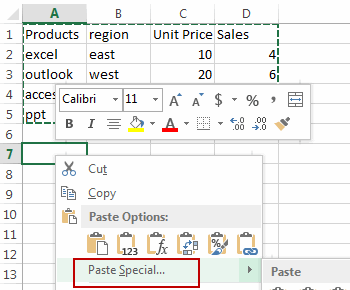#4 select Transpose checkbox in the Paste Special dialog box. And click OK button.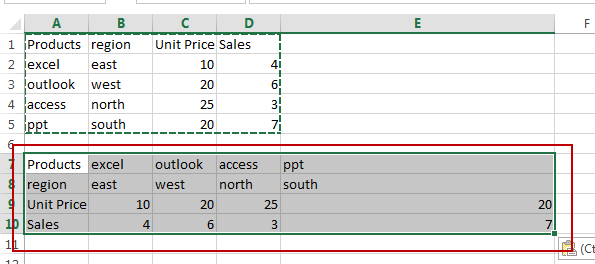## Convert Rows to Columns Using Formula

You can also use a formula based on the TRANSPOSE function to quickly convert columns to rows in Excel. Here are the steps:

#1 you need to count the number of rows and columns in your range A1:D5 and select the same number of blank cells (4 rows and 5 columns)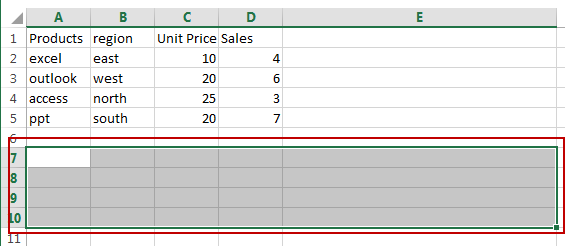#2 type the following formula in the first empty cell of your selected range of cells.

`=TRANSPOSE(A1:D5)`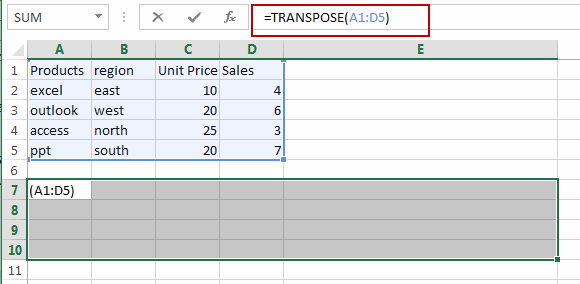#3 then press Ctrl + Shift + Enter keys on your keyboard to make it an Excel Array formula. You would notice that the columns are converted to rows for your original data A1:D5.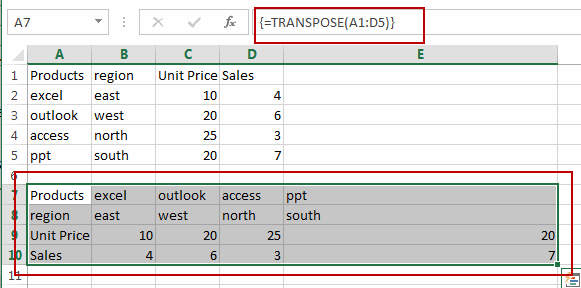## Convert Rows to Columns Using VBA

You can also use an Excel VBA Macro to achieve the same result of converting rows to columns. Just do the following steps:

#1 open your excel workbook and then click on “Visual Basic” command under DEVELOPER Tab, or just press “ALT+F11” shortcut.#2 then the “Visual Basic Editor” window will appear.

#3 click “Insert” ->”Module” to create a new module.#4 paste the below VBA code  into the code window. Then clicking “Save” button.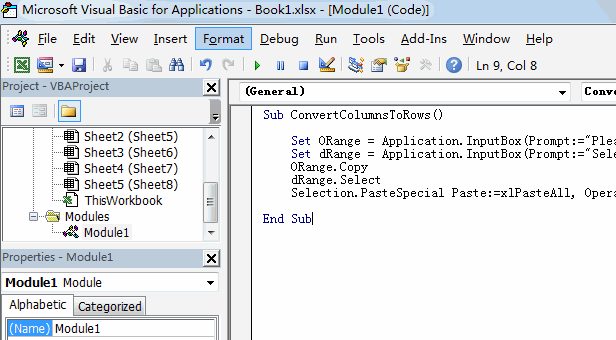```Sub ConvertColumnsToRows()
Set ORange = Application.InputBox(Prompt:="Please select one range that you want to transpose", Title:="ConvertColumnsToRows", Type:=8)
Set dRange = Application.InputBox(Prompt:="Select the first cell of the destination range", Title:="ConvertColumnsToRows", Type:=8)
ORange.Copy
dRange.Select
Selection.PasteSpecial Paste:=xlPasteAll, Operation:=xlNone, SkipBlanks:=False, Transpose:=True
End Sub```

#5 back to the current worksheet, then run the above excel macro. Click Run button.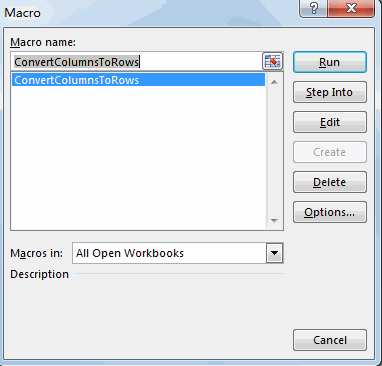#6 please select one range that you want to transpose. Click OK button.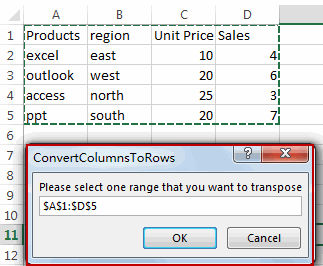#7 select the first cell of the destination range. Click OK button.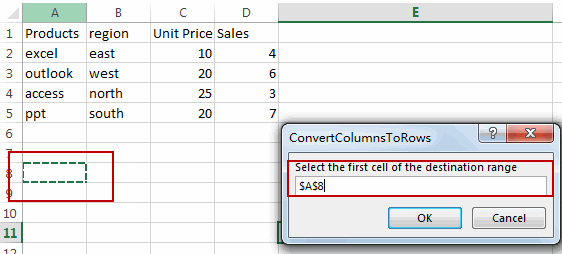#8 back to the current worksheet, then run the above excel macro. Click Run button.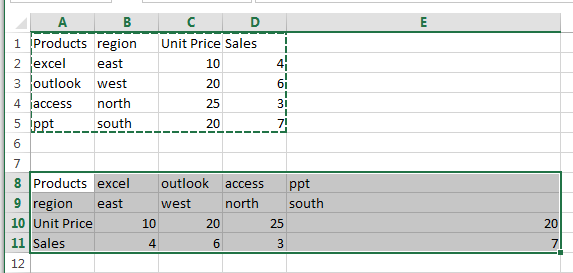### Related Functions

• Excel TRANSPOSE function
Excel TRANSPOSE formula allows you to rotate (swap) values from rows to columns and vice versa in Excel.The Excel TRANSPOSE Function syntax:=TRANSLATE (range) …
Related Posts

Find and Replace Multiple Values

This post will guide you how to find and replace multiple values at once with VBA macro or using formula  in Excel. How do I make multiple find and replace in Excel. Suppose that you have a few cells containing ...

VBA Macro For VLOOKUP From Another Sheet

In the previous post, you should know that how to fix or remove the #N/A error when using VLOOKUP formula to lookup value from another sheet. And this post will show you how to use VBA code to vlookup data ...

How To Insert Comments in Protected Worksheet in Excel

This post will show you how to allow comments in a protected worksheet in Excel. You can easily to insert comments into cells in a normal worksheet in Excel, but if want to insert a comment in a worksheet that ...

How To Convert Text to Upper Cases(Using VBA) in Excel

This post will show you how to switch from lower case to upper case in Excel. and I am going to show you two different ways of converting text to upper cases using formula or VBA macro in Excel 2013,Excel ...

How To Hide Every Other Row in Excel (Using VBA)

This post will show you how to hide alternate rows or columns in Excel or how to hide every third, fourth, fifth row or column in Excel. If you want to hide every other row in your current worksheet, how ...

Sidebar